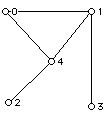Partitions and Eccentricities and Degrees of Vertices in a Graph

Prepared by:

Joseph Malkevitch
Department of Mathematics and Computer Studies
York College (CUNY)
Jamaica, New York

email:

malkevitch@york.cuny.edu

web page:

http://york.cuny.edu/~malk

Suppose G is a connected graph. If two vertices of G are u and v then the distance between u and v is the number of edges in a shortest path from u to v. The eccentricity of a vertex v in a connected graph is equal to the distance between and some vertex w so that the distance between v and w is as large as possible. The eccentricity of a vertex v is the farthest that any vertex can be from v. I will use the notation e(v) to denote the eccentricity of v. Also of interest for the vertices of a graph are their degrees or valences. The valence or degree of v, denoted d(v), will be the number of local line segments that meet at v. (This definition allows one to use loops in the graph, however, for simplicity here I will consider only graphs without loops or multiple edges. Also that note there are special types of connected graphs of interest: trees; 2-connected, planar; planar 3-connected, etc.

Figure 1 shows an example of a connected planar graph.Figure 1

For each vertex we can write down its valence and eccentricity. Thus,
e(0) = 2, d(0) =2; e(1) = 2, d(1) = 3; e(2) = 3, d(2)=1; e(3) = 3, d(3) = 1; e(4) = 2, d(4) = 3.

Thus, for this graph we have 3 vertices of eccentricity 2 and 2 vertices of eccentricity 3 and 2 vertices of degree 1, 2 vertices of degree 3 and 1 vertex of degree 2. So, this graph illustrates a graph with 5 vertices of partition type {3, 2} for eccentricities and partition type {2, 2, 1} for degrees. We can assign this graph a partition type based on pairs - the first entry of the pair is for eccentricity, and the second for degrees. In this case the graph in Figure 1 is of type {3, 2}; {2, 2, 1}. We can raise the general questions based on what we see here.

Question 1

For connected graphs with n vertices what eccentricity partition types are possible?

Special cases: trees, planar graphs, planar 3-connected graphs

Question 2

For connected graphs with n vertices what degree partition types are possible?

Special cases: trees, planar graphs, planar 3-connected graphs

Question 3

For connected graphs with n vertices what eccentricity-degree pairs of partition types are possible?

Special cases: trees, planar graphs, planar 3-connected graphs

Other approaches to this circle of ideas is possible including "order" issues.

Note: The answer for small values of n may be special and more general things may be noticeable as n grows.